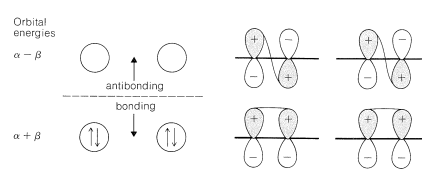# 21.5: Application of the MO Method to 1,3-Butadiene

$$\newcommand{\vecs}{\overset { \rightharpoonup} {\mathbf{#1}} }$$ $$\newcommand{\vecd}{\overset{-\!-\!\rightharpoonup}{\vphantom{a}\smash {#1}}}$$$$\newcommand{\id}{\mathrm{id}}$$ $$\newcommand{\Span}{\mathrm{span}}$$ $$\newcommand{\kernel}{\mathrm{null}\,}$$ $$\newcommand{\range}{\mathrm{range}\,}$$ $$\newcommand{\RealPart}{\mathrm{Re}}$$ $$\newcommand{\ImaginaryPart}{\mathrm{Im}}$$ $$\newcommand{\Argument}{\mathrm{Arg}}$$ $$\newcommand{\norm}{\| #1 \|}$$ $$\newcommand{\inner}{\langle #1, #2 \rangle}$$ $$\newcommand{\Span}{\mathrm{span}}$$ $$\newcommand{\id}{\mathrm{id}}$$ $$\newcommand{\Span}{\mathrm{span}}$$ $$\newcommand{\kernel}{\mathrm{null}\,}$$ $$\newcommand{\range}{\mathrm{range}\,}$$ $$\newcommand{\RealPart}{\mathrm{Re}}$$ $$\newcommand{\ImaginaryPart}{\mathrm{Im}}$$ $$\newcommand{\Argument}{\mathrm{Arg}}$$ $$\newcommand{\norm}{\| #1 \|}$$ $$\newcommand{\inner}{\langle #1, #2 \rangle}$$ $$\newcommand{\Span}{\mathrm{span}}$$$$\newcommand{\AA}{\unicode[.8,0]{x212B}}$$

To treat the $$\pi$$-electron system of 1,3-butadiene by simple MO theory, we combine the four $$p$$ carbon orbitals of an atomic-orbital model, such as $$17$$, to obtain four molecular orbitals:Figure 21-8). Therefore the delocalization energy is $$\left( 4 \alpha + 4.48 \beta \right) - \left( 4 \alpha + 4 \beta \right) = 0.48 \beta$$ or $$9 \: \text{kcal}$$, assuming that $$\beta = 19 \: \text{kcal}$$. (The corresponding VB calculation gives the delocalization in good agreement as $$0.23 J$$ or $$8 \: \text{kcal}$$; Section 21-3D.)Figure 21-7: Energies and schematic representations of the $$\pi$$ molecular orbitals of 1,3-butadiene. If four electrons are placed in the two lowest orbitals, the $$\pi$$-electron energy is $$2 \left( \alpha + 1.62 \beta \right) + 2 \left( \alpha + 0.62 \beta \right) = 4 \alpha + 4.48 \beta$$. The schematic representations show the number of phase changes (nodes) in each molecular orbital, and the sizes of the atomic orbitals are drawn to represent crudely the extent to which each contributes to each molecular orbital. Again, the energy of the orbitals increases with increasing number of nodes.

We can estimate a stabilization energy for butadiene from heats of hydrogenation, and it is useful to compare the values obtained with the calculated delocalization energy. Thus the heat of hydrogenation of 1,3-butadiene is $$57.1 \: \text{kcal}$$, whereas that of ethene is $$32.8 \: \text{kcal}$$ and of propene $$30.1 \: \text{kcal}$$. If ethene is used as the model alkene, the stabilization energy of 1,3-butadiene is $$\left( 2 \times 32.8 - 57.1 \right) = 8.5 \: \text{kcal}$$, whereas with propene as the model, it would be $$\left( 2 \times 30.1 - 57.1 \right) = 3.1 \: \text{kcal}$$. The bond energies (Table 4-3) in combination with the heat of formation at $$25^\text{o}$$ $$\left( 26.33 \: \text{kcal} \right)$$ give a stabilization energy of $$5.0 \: \text{kcal}$$.Figure 21-8: Energies and schematic representations of the $$\pi$$ molecular orbitals of localized 1,3-butadiene. The orbitals are the $$\pi$$ orbitals of two isolated ethene bonds and the total $$\pi$$-electron energy is $$4 \left( \alpha + \beta \right) = 4 \alpha + 4 \beta$$.